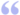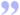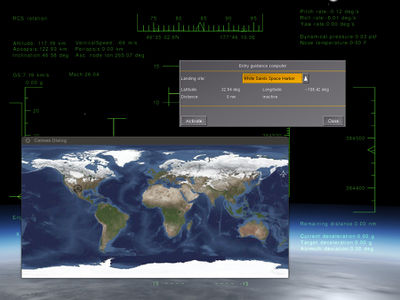Hi fellow wiki editors!

To help newly registered users get more familiar with the wiki (and maybe older users too) there is now a {{Welcome to the wiki}} template. Have a look at it and feel free to add it to new users discussion pages (and perhaps your own).

I have tried to keep the template short, but meaningful. /Johan G

# Difference between revisions of "Talk:Canvas Snippets"

## Documenting supported options/parametersyou made a good point that we should probably document supported parameters/attributes or "modes" whenever possible. I think it would make sense to extend the underlying template accordingly and provide a list of documented options. — Hooray (Fri Apr 10). Improving Canvas docs (cont'd PM). (powered by Instant-Cquotes)## Space Shuttle SnippetsOkay, I have a nice loaded earth texture picture. I have the position of my shuttle: first thing I want is to draw it in real time on the picture, see it move. Next thing is - I want to display the ground track. What do I do - store past positions into an array regularly? Is it done automatically? Beyond that, I want to display the groundtrack prediction based on current state. So, I can create an array of future points. Or properties. Or pass the function which, when given a time, will spit out coordinates. Don't care - whatever is best. Finally, I'd like to have the ability to display the selected landing site on the map. But that's probably the same as the shuttle problem - I have a set of coords and I want a symbol drawn there. Probably all are solved problems - but how? — Thorsten (Fri Apr 10). Re: Using Canvas for visualizing orbital flights (cont'd PM). (powered by Instant-Cquotes)```var (width,height) = (800,400);

# create a new window, dimensions are 400 x 400, using the dialog decoration (i.e. titlebar)
var window = canvas.Window.new([width, height],"dialog");

# adding a canvas to the new window and setting up background colors/transparency
var myCanvas = window.createCanvas().set("background", canvas.style.getColor("bg_color"));

# creating the top-level/root group which will contain all other elements/group
var root = myCanvas.createGroup();

# path is now a URL (could also be stored in \$FG_ROOT and be fixed path instead)
var url = "http://www.worldwidetelescope.org/docs/Images/MapOfEarth.jpg";

# this would need to be changed when using a different image
var map_width = 310;
var map_height = 155;

var x_offset = (width-map_width)/2;
var y_offset = (height-map_height)/2;

# assuming Mercator projection for now (untested pseudo code)
var position2Pixels = func(lat, lon) {
var xpos = (lon+180)*(map_width/360);
var ypos= (map_height/2)-(map_width*mercN/(2*math.PI));

return [xpos+x_offset, ypos+y_offset];
}

# create an image child for the texture
var child=root.createChild("image")
.setFile( url )
.setTranslation( x_offset, y_offset ) # centered, in relation to dialog coordinates
.setSize(image_width, image_height); # image dimensions```

### Adding a dynamically positioned symbol

 Note  This snippet is generic enough to be usable for both use-cases: showing a moving aircraft/shuttle symbol, but also adding a static landing site. However, you'd obviously not update static symbols each frame using a timer.
```# this could also be any other SVG file
var svg_path = "Nasal/canvas/map/Images/boeingAirplane.svg";

# create an empty Canvas group for holding the space shuttle symbol
var shuttle_symbol = root.createChild("group", "shuttle-symbol");

# parse the SVG file
# this converts the SVG file into OpenVG instructions
# supported by Canvas

canvas.parsesvg(svg_symbols, svg_path);

# resize the SVG image
shuttle_symbol.setScale(0.20); # 20%

var update_shuttle = func() {
var lat = getprop('/position/latitude-deg');
var lon = getprop('/position/longitude-deg');

var (x,y) = position2Pixels(lat:lat,lon:lon);
shuttle_symbol.setTranslation(x,y,);
}

# can use maketimer() to call this regularly
update_shuttle();```

### Adding a dynamically computed line/curve

`var groundtrack = root.createChild("path");`

## Orbital Mapthis is probably rather crude, but it (sort of) works - I'm getting the current position of the shuttle as well as the selected landing site displayed. — Thorsten (Sat Apr 11). Re: Using Canvas for visualizing orbital flights (cont'd PM). (powered by Instant-Cquotes)In case you people are still interested - this may be useful (world map with historic and predicted groundtrack, showing landing site and spacecraft position) and could be adapted to your mission control. Code is posted below, you need to supply inclination and orbital period - just use standard orbital dynamics formulae from Wikipedia. No warranties. — Thorsten (Wed Apr 15). Re: OpenRadar special use for especific space missions. (powered by Instant-Cquotes)```var sym_shuttle = {};
var sym_landing_site = {};

var (width,height) = (800,400);

var graph = {};
var samples = [];
var history = [];
var track_prediction = [];

var update_loop_flag = 0;

var lat_to_m = 110952.0; # latitude degrees to meters
var m_to_lat = 9.01290648208234e-06; # meters to latitude degrees
var lon_to_m = 0.0; # needs to be calculated dynamically
var m_to_lon = 0.0; # we do this on startup

var delete_from_vector = func(vec, index) {

var n = index+1;

var vec_end = subvec(vec, n);

setsize(vec, n-1);
return vec~vec_end;
}

var calc_geo = func(lat) {

lon_to_m  = math.cos(lat*math.pi/180.0) * lat_to_m;
m_to_lon = 1.0/lon_to_m;
}

var lat_to_y = func (lat) {

return height/2 - lat /90. * height/2;

}

var lon_to_x = func (lon) {

return width/2 + lon /180. * width/2;

}

var create_map = func {

var window = canvas.Window.new([width,height],"dialog").set("title", "Trajectory Map");

# we need to explicitly re-define this to get a handle to stop the update loop
# upon closing the window

window.del = func()
{
#print("Cleaning up...\n");
update_loop_flag = 0;
call(canvas.Window.del, [], me);
};

var mapCanvas = window.createCanvas().set("background", canvas.style.getColor("bg_color"));

var root = mapCanvas.createGroup();

var path = "Aircraft/SpaceShuttle/Dialogs/MapOfEarth.png";
var child=root.createChild("image")
.setFile( path )
.setTranslation(0,0)
.setSize(width,height);
sym_shuttle = mapCanvas.createGroup();
canvas.parsesvg(sym_shuttle, "/Nasal/canvas/map/Images/boeingAirplane.svg");
sym_shuttle.setScale(0.2);

sym_landing_site = mapCanvas.createGroup();
canvas.parsesvg(sym_landing_site, "/gui/dialogs/images/ndb_symbol.svg");
sym_landing_site.setScale(0.6);

graph = root.createChild("group");

update_loop_flag = 1;
map_update();

}

var map_update = func {

if (update_loop_flag == 0 ) {return;}

var lat = getprop("/position/latitude-deg");
var lon = getprop("/position/longitude-deg");

var x =  lon_to_x(lon);
var y =  lat_to_y(lat);

sym_shuttle.setTranslation(x,y);

x = lon_to_x(landing_site.lon()) - 10.0;
y = lat_to_y(landing_site.lat()) - 10.0;

sym_landing_site.setTranslation(x,y);

prediction_update();
plot_tracks();

settimer(map_update, 1.0);

}

var plot_tracks = func  {

graph.removeAllChildren();
var plot = graph.createChild("path", "data")
.setStrokeLineWidth(2)
.setColor(0,0,1)
.moveTo(history,history);

for (var i = 1; i< (size(history)-1); i=i+1)
{
var set = history[i+1];
if (history[i+1] > history[i])
{
plot.lineTo(set, set);
}
else
{
plot.moveTo(set, set);
}
}

var pred_plot = graph.createChild("path", "data")
.setStrokeLineWidth(2)
.setColor(1,0,0)
.moveTo(track_prediction,track_prediction);

for (var i = 1; i< (size(track_prediction)-1); i=i+1)
{
var set = track_prediction[i+1];
if (track_prediction[i+1] > track_prediction[i])
{
pred_plot.lineTo(set, set);
}
else
{
pred_plot.moveTo(set, set);
}
}

}

var history_init = func {

var lat = getprop("/position/latitude-deg");
var lon = getprop("/position/longitude-deg");
var x =  lon_to_x(lon);
var y =  lat_to_y(lat);

for (var i = 0; i < 1000; i = i+1)
{
var set = [x,y];
append(history,set);
}
history_update();

}

var history_update = func {

history = delete_from_vector(history,0);

var lat = getprop("/position/latitude-deg");
var lon = getprop("/position/longitude-deg");
var x =  lon_to_x(lon);
var y =  lat_to_y(lat);

append(history, [x,y]);

settimer(history_update, 10.0);
}

var prediction_update = func {

setsize(track_prediction,0);

var earth_motion_degs = 0.00416666666;
var lat = getprop("/position/latitude-deg");
var lon = getprop("/position/longitude-deg");

calc_geo(lat);

var orbiter_motion_north_fps = getprop("/fdm/jsbsim/velocities/v-north-fps");

var orbital_speed_fps = getprop("/fdm/jsbsim/velocities/eci-velocity-mag-fps");
var orbital_period = getprop("/fdm/jsbsim/systems/orbital/orbital-period-s");

var rising_flag = 0.0;

if (orbiter_motion_north_fps > 0.0) {rising_flag = 1;}
else {rising_flag = -1.0};

var inclination = getprop("/fdm/jsbsim/systems/orbital/inclination-deg");
var inclination_rad = inclination * math.pi/180.0;

var sinphi = (lat/ inclination);

sinphi = math.min(sinphi, 1.0);
sinphi = math.max(sinphi,-1.0);

var phi = math.asin(sinphi);

if (rising_flag == -1) {phi = math.pi - phi;}

var lon_rising = lon - phi * 180.0/math.pi;
if (lon_rising < 0.0) {lon_rising = lon_rising + 360.0;}

var dt =  120.0;
var offset = 0.0;

var increment = 2.0 * math.pi * dt/orbital_period;

for (var i = 0; i<40; i = i+1)
{
var arg = phi + i * increment;

var pred_lat = math.asin(math.sin(arg) * math.sin(inclination_rad));
var pred_lon = math.atan2(math.cos(inclination_rad) * math.sin(arg), math.cos(arg));

pred_lat = pred_lat * 180.0/math.pi;
pred_lon = pred_lon * 180.0/math.pi;

pred_lon = pred_lon + lon_rising - earth_motion_degs * i * dt;

if (i==0)
{
offset = lon - pred_lon;
}
pred_lon = pred_lon + offset;

if (pred_lon > 180) {pred_lon = pred_lon - 360;}
if (pred_lon < -180) {pred_lon = pred_lon + 360.0;}
var x =  lon_to_x(pred_lon);
var y =  lat_to_y(pred_lat);
append(track_prediction, [x,y]);
}

}

history_init();```

## extending the snippet template

• required minimal FG version
• external dependencies (files)
• note/caution if snippet has been updated and not yet tested
• related docs

## checkbox snippet missing

need to add snippet showing use of checkboxes

## Drag & Drop snippet

```var (width,height) = (640,480);
var title = 'Canvas Drag & Drop demo';

# create a new window, dimensions are WIDTH x HEIGHT, using the dialog decoration (i.e. titlebar)
var window = canvas.Window.new([width,height],"dialog")
.set('title',title);

# adding a canvas to the new window and setting up background colors/transparency
var myCanvas = window.createCanvas().set("background", canvas.style.getColor("bg_color"));

# Using specific css colors would also be possible:
# myCanvas.set("background", "#ffaac0");

# creating the top-level/root group which will contain all other elements/group
var root = myCanvas.createGroup();

##############

# http://wiki.flightgear.org/Canvas_Event_Handling
var setupEventHandler = func(element) {

var path = element.get('src');
# http://wiki.flightgear.org/Tooltips
gui.popupTip("Filename is:"~path);

}); # mouseover event handler

element.setTranslation(event.clientX, event.clientY);
}); # drag event

}

var images = ['Splash1.png','Splash2.png','Splash3.png'];

var (width, height) = (150,150);

foreach(var img; images) {

# create an image child for the texture
var newImage = root.createChild("image")
.setFile("Textures/"~ img)
.setTranslation(xOffset, 10)
.setSize(width, height);

setupEventHandler(element: newImage);